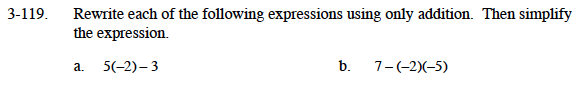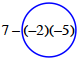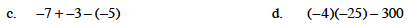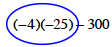### Home > MC1 > Chapter 3 > Lesson 3.4.3 > Problem3-119

3-119.
1. . Rewrite each of the following expressions using only addition. Then simplify the expression. Homework Help ✎

1. 5(−2) − 3

2. 7 − (−2)(−5)

3. −7 + −3 − (−5)

4. (−4)(−25) − 3005(−2)+(−3) = −13

Circling terms will also be helpful in this problem.The equivalent addition expression is −7+(−3)+5. Remember to simplify.

Circling terms first will be helpful. This has been done for you.